1593217200

# Depth First — In Order Algorithm Visually Explained

There are three types of depth first binary tree traversals:

• Pre-order:
• In-order:
• Post-order:

We will be focusing on the In-Order Binary Tree Traversal. We can see that starting from the root node, we’ll visit all the nodes in the left subtree, come back to the root node and then visit all the vertices in the right subtree.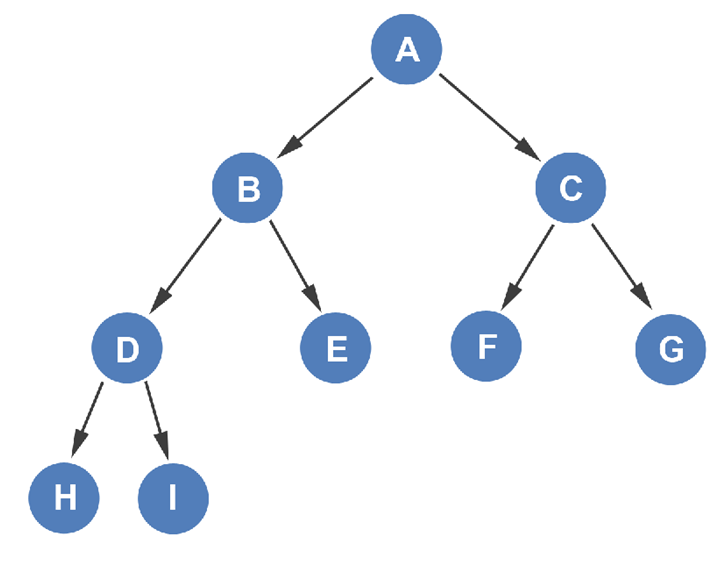Next to each node, we’ll put a couple of reminders for ourselves:

• left subtree
• visit/print node
• right subtree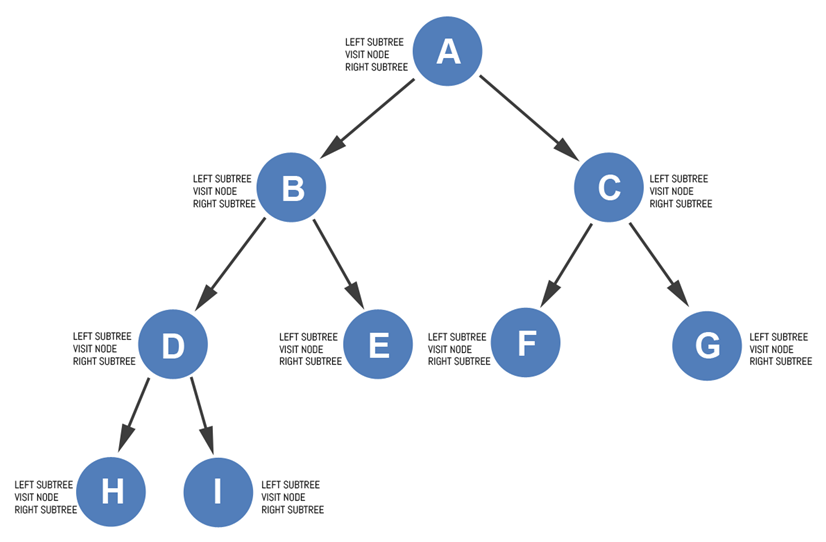We’ll begin with the root node.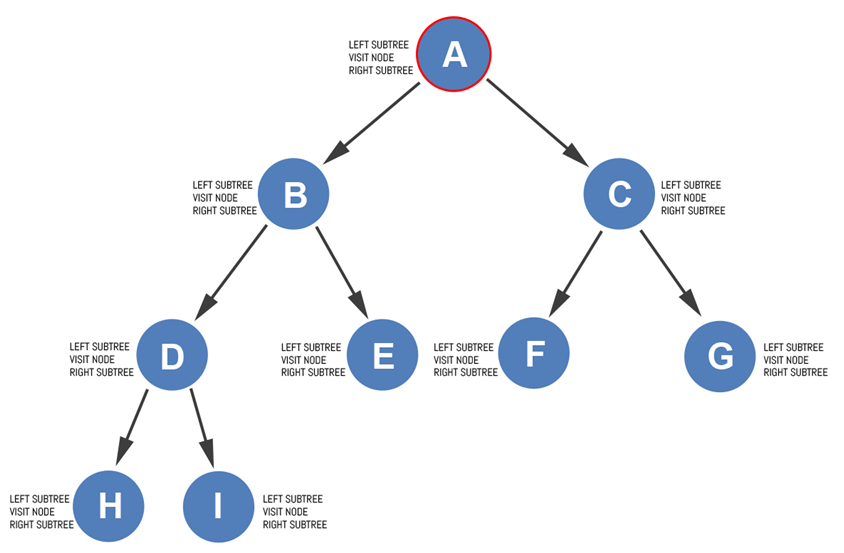At this point, we can cross out the left subtree label and start moving down the left subtree.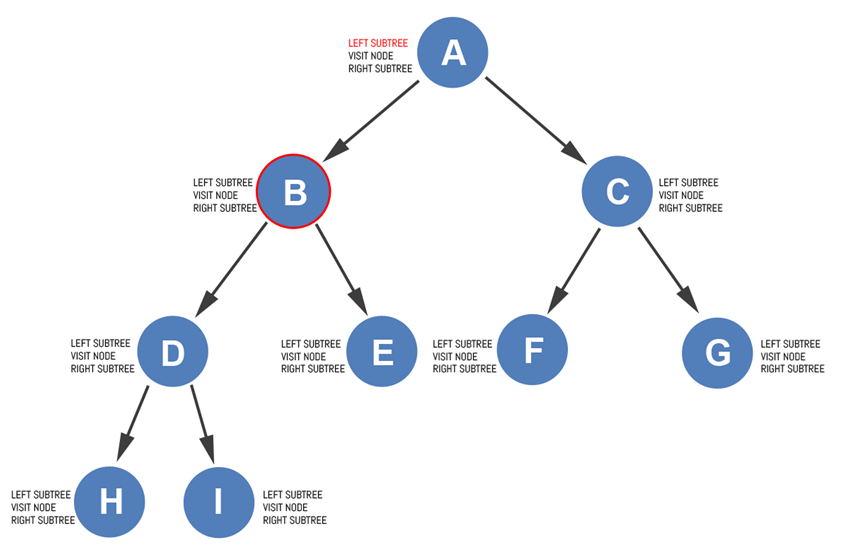Since vertex B also has a left subtree, according to the rules outlined by in-order traversal (), we must visit its left subtree next. So, we cross out the left subtree label on vertex B and move to vertex D.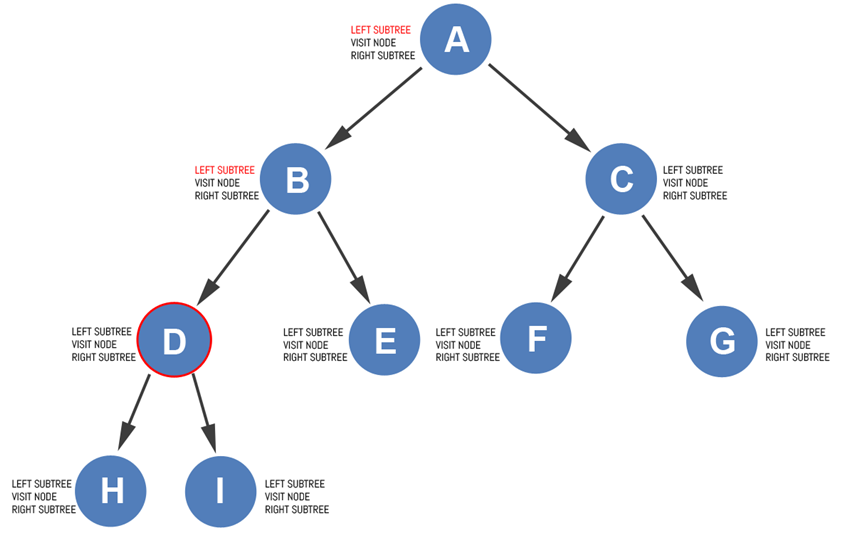Vertex D also has a left subtree, so we visit its left subtree next. We cross out the left subtree label on vertex D and move to vertex H.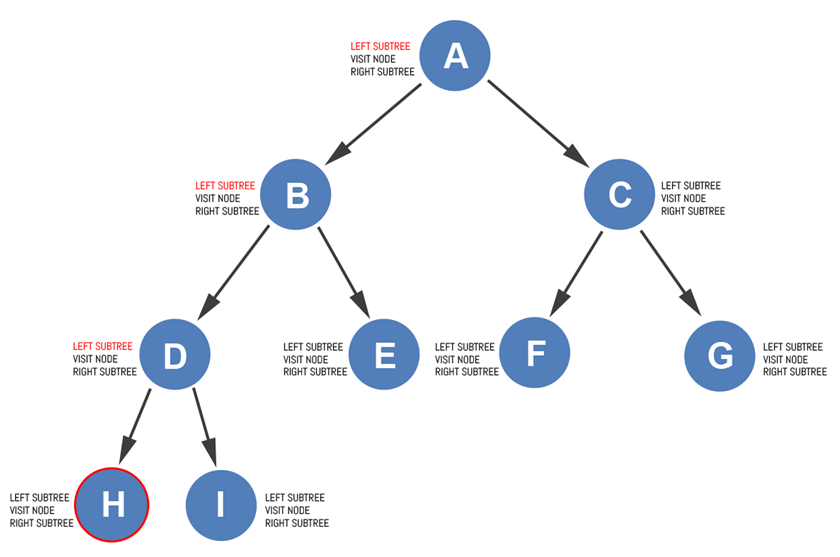We can quickly see that vertex H does not have any other left subtrees. We can safely cross that out.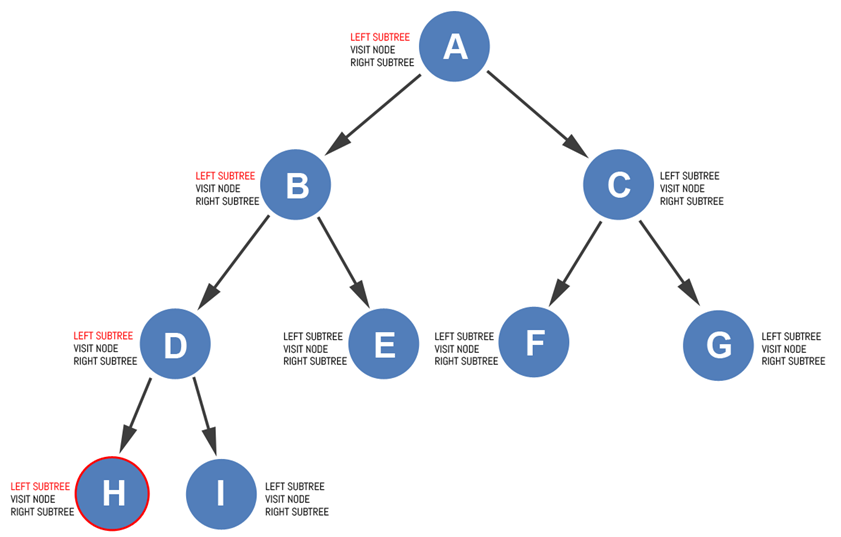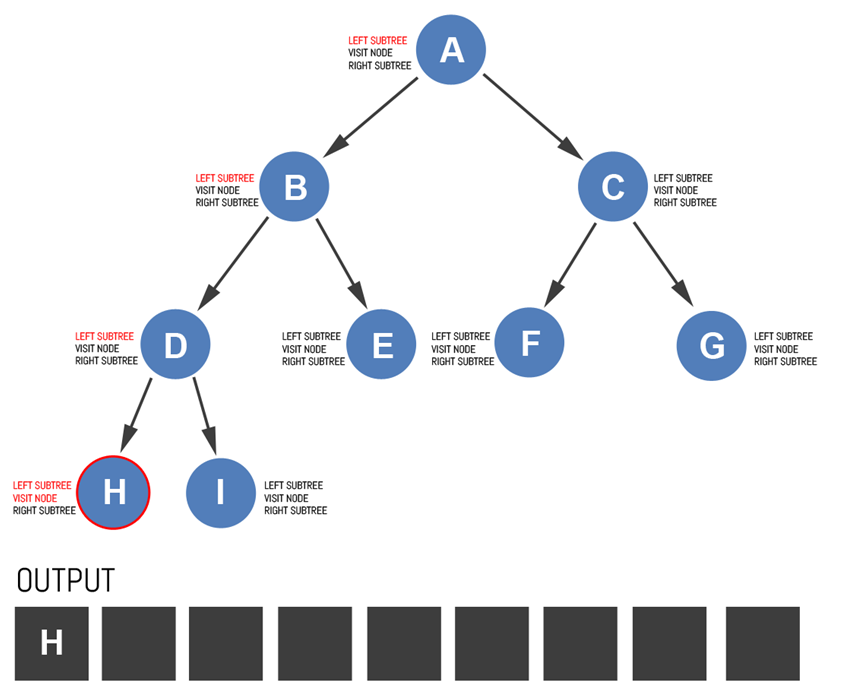An attempt is made to visit node H’s right subtree, but vertex H has no right subtree. The right subtree label is crossed out and we return to node D. We are now visiting node D after visiting its left subtree. We can add node D to the output.

#computer-science #programming #binary-tree #algorithms #binary-tree-traversal

## Buddha Community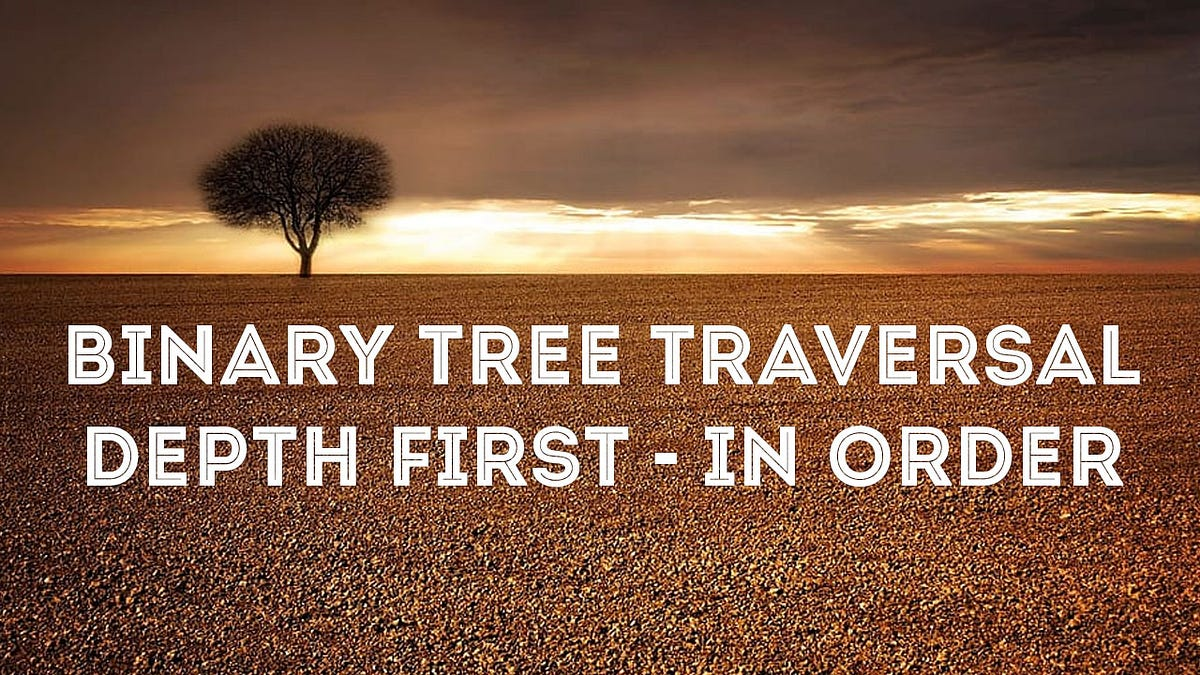1592844780

## Depth First Search (DFS) Algorithm Visually Explained

The Depth First Search algorithm traverses the graph and explores each adjacent node before backtracking and moving to the next node. It utilizes the stack data structure. Remember, the stack uses the last-in-first-out (LIFO) approach. We push items onto the stack and pop items from the top of the stack. We’ll keep track of the stack and we’ll show the vertices in the order of which they were discovered.
We’ll begin our traversal at vertex A. Vertex A has been discovered, so it’s added to the discovery list. Vertex A is also pushed onto the stack and is marked as visited.

#dfs #algorithms #programming #depth-first-search #computer-science1593217200

## Depth First — In Order Algorithm Visually Explained

There are three types of depth first binary tree traversals:

• Pre-order:
• In-order:
• Post-order:

We will be focusing on the In-Order Binary Tree Traversal. We can see that starting from the root node, we’ll visit all the nodes in the left subtree, come back to the root node and then visit all the vertices in the right subtree.Next to each node, we’ll put a couple of reminders for ourselves:

• left subtree
• visit/print node
• right subtreeWe’ll begin with the root node.At this point, we can cross out the left subtree label and start moving down the left subtree.Since vertex B also has a left subtree, according to the rules outlined by in-order traversal (), we must visit its left subtree next. So, we cross out the left subtree label on vertex B and move to vertex D.Vertex D also has a left subtree, so we visit its left subtree next. We cross out the left subtree label on vertex D and move to vertex H.We can quickly see that vertex H does not have any other left subtrees. We can safely cross that out.An attempt is made to visit node H’s right subtree, but vertex H has no right subtree. The right subtree label is crossed out and we return to node D. We are now visiting node D after visiting its left subtree. We can add node D to the output.

#computer-science #programming #binary-tree #algorithms #binary-tree-traversal1616572311

## Originscale Order Management System

Originscale order management software helps to manage all your orders across channels in a single place. Originscale collects orders across multiple channels in real-time - online, offline, D2C, B2B, and more. View all your orders in one single window and process them with a simple click.

#order management system #ordering management system #order management software #free order management software #purchase order management software #best order management software1591177440

## Visual Analytics and Advanced Data Visualization

Visual Analytics is the scientific visualization to emerge an idea to present data in such a way so that it could be easily determined by anyone.

It gives an idea to the human mind to directly interact with interactive visuals which could help in making decisions easy and fast.

Visual Analytics basically breaks the complex data in a simple way.

The human brain is fast and is built to process things faster. So Data visualization provides its way to make things easy for students, researchers, mathematicians, scientists e

#blogs #data visualization #business analytics #data visualization techniques #visual analytics #visualizing ml models1626268740

## Depth First Search (DFS) in Data Structure

In the last article, we learned about graphs in data structures. Graphs are one of the efficient ways that are used to model daily life problems and find an optimal solution. In this article, we will learn about traversing techniques for the graph and their implementation

#### Depth First Search

DFS is a recursive traversal algorithm for searching all the vertices of a graph or tree data structure. It starts from the first node of graph G and then goes to further vertices until the goal vertex is reached.

• DFS uses stack as its backend data structure
• edges that lead to an unvisited node are called discovery edges while the edges that lead to an already visited node are called block edges.

#### DFS procedure

DFS implementation categorizes the vertices in the graphs into two categories:

• Visited
• Not visited

The major objective is to visit each node and keep marking them as “visited” without making any cycle.

#### Steps for DFS algorithms:

1. Start by pushing starting vertex of the graph into the stack

2. Pop the top item of the stack and add it to the visited list

3. Create the adjacency list for that vertex. Add the non-visited nodes in the list to the top of the stack

4. Keep repeating steps 2 and 3 until the stack is empty

#### Depth First Search Algorithm

• Step 1: STATUS = 1 for each node in Graph G
• Step 2: Push the starting node A in the stack. set its STATUS = 2
• Step 3: Repeat Steps 4 and 5 until STACK is empty
• Step 4: Pop the top node N from the stack. Process it and set its STATUS = 3
• Step 5: Push all the neighbors of N with STATUS =1 into the stack and set their STATUS = 2
• [END OF LOOP]
• Step 6: stop

#data structure tutorials #applications of depth first search #depth first search #data structure